Maths-
General
Easy

Question

# The number of ways in which 17 billiard balls be arranged in a row if 7 of them are black, 6 are red, 4 are white is

##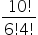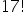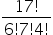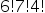Hint:

## The correct answer is:### Detailed SolutionBefore solving the question, we are going to assume that all the balls of the same color are identical. This means that one red ball is identical to another red ball. Similarly, one black ball is identical to all the other black balls and one white ball will be similar to other white balls. Now, we are given that out of 17 balls, 7 of them are black, 6 are red and 4 are white. Now, we will arrange these balls in a row. The formula by which we can arrange the total number of entities which contain similar entities is given as:Thus, we get,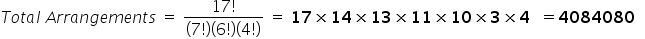Thus, there are 4084080 ways in which we can arrange these billiard balls.

We cannot arrange the billiards balls as follows:
There are 17 balls, so the total number of arrangements = 17!
This is incorrect because this method is applicable only when the balls are distinct, not identical. But, in our case, all the balls of the same color are identical.#### With Turito Foundation.#### Get an Expert Advice From Turito.